Vocabulary
Rules
One Transformation
Series of Transformations
Identify the Transformation
100

What does congruent mean?

The same or equal to

100

What is the rule for sliding a figure 3 units right and 2 units down?

(x + 3, y - 2)

100

Translate the triangle ABC along the vector <3, -2>.

A (0,2)

B (1,4)

C (5,-2)

A' (3, 0)

B' (4, 2)

C' (8, -4)

100

Reflect the line segment XY where X (2, 3) and Y (4, -2) over the x - axis, then dilate using a scale factor of 2.

X' (4. -6)

Y' (8, 4)

100

Identify the transformation.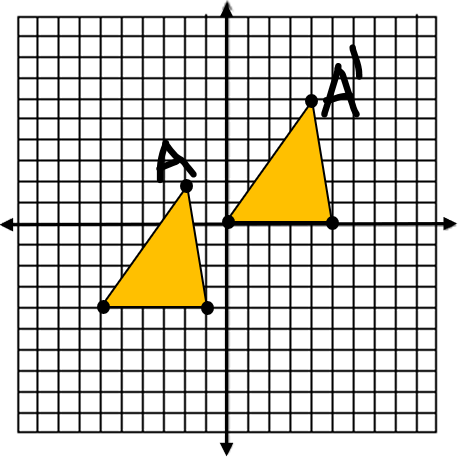Translation

*BONUS 100 points: State the translation rule used in the graph.

200

What is it called when the preimage and the image are congruent?

Isometry

200

How do you dilate a figure?

Multiply by a scale factor

200

Reflect the points across the y-axis

A (1,2)

B (1,4)

C (5,-2)

A' (-1,2)

B' (-1,4)

C' (-5,-2)

*BONUS 100 points: Reflect the image across the x-axis and state the points as A" B" C"

200

Translate the figure 3 units left and 4 down, then rotate 90 C around the origin.

A (-1, 3) B (1, 2) C (0, -1)

A' (-1, 4)

B' (-2, 2)

C' (-5, 3)

200

Identify the transformation.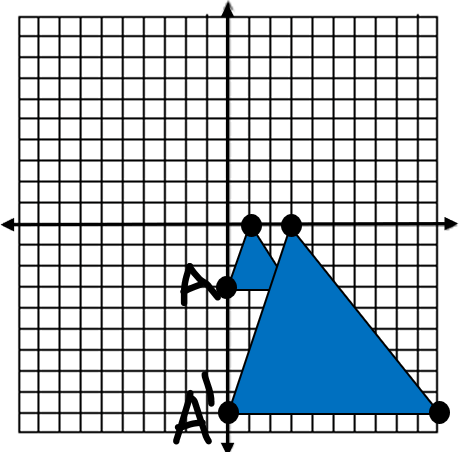Dilation

*BONUS 100 points: What scale factor was used in this dilation?

300

What is a preimage?

Preimage: the figure before the transformation

300

What is the rule for rotating 270 counterclockwise?

(x, y) --> (y, -x)

300

Rotate the points about the origin by 90 C.

A (2,4)

B (-1,0)

C (6,1)

A' (4, -2)

B' (0, 1)

C' (1, -6)

300

Identify the series of transformations.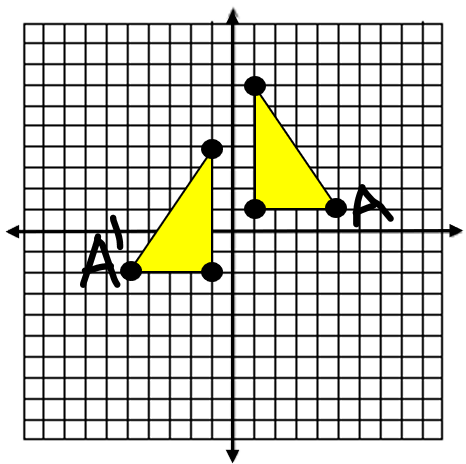1. reflection over the y-axis

(x, y) --> (-x, y)

2. slide 3 units down

(x, y) --> (x, y - 3)

300

Identify this transformation.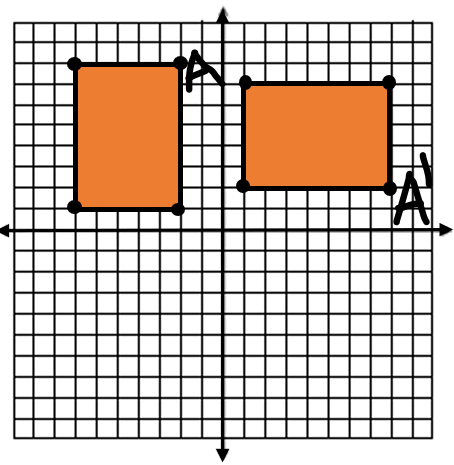Rotate 90 clockwise

*BONUS 100 points: what is the rule for rotating 90 clockwise?

400

What are the key words for the following transformations:

1. translation

2. reflection

3. rotation

4. dilation

1. slide

2. flip

3. turn

4. size

400

State the rule for reflecting over the line y = x.

(x, y) --> (y, x)

*switch the x- and y-values

400

Dilate the points about the origin using a scale factor of 3.

A (2,4)

B (-1,0)

C (6,1)

A' (6, 12)

B' (-3, 0)

C' (18, 3)

400

Translate the points along the vector <3,2>, then reflect over the line y = -x.

A (1, 2)

B (3, 7)

C (6, 2)

A' (-4, -4)

B' (-9,  -6)

C' (-4, -9)

400

Identify the transformation.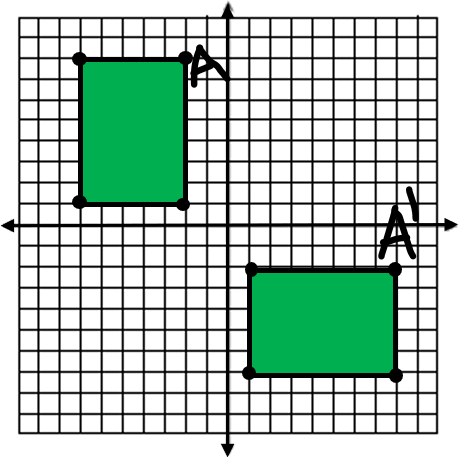Reflection over the line y = x

*BONUS 100 points: What is the rule for reflecting over the line y = x?

500

What is a vertex?

A point of a figure

*100 bonus - What is the word if there is more than one vertex?

500

What are the steps for rotating a figure around a point other than the origin?

1. subtract the center of rotation

2. apply the rotation rule

3. add the center of origin

500

Rotate the points 90 CC around the point (3, 2).

A (2, 4)

B (-1, 0)

C (6, 1)

A' (1, 1)

B' (5, -2)

C' (4, 5)

500

Dilate using a scale factor of  1/3 , then rotate 90 CC around (3, 2).

X (12, -6) Y (9, 3) Z (-3, -9)

X' (7, 3)

Y' (2, 2)

Z' (8, -2)

500

Identify the transformation.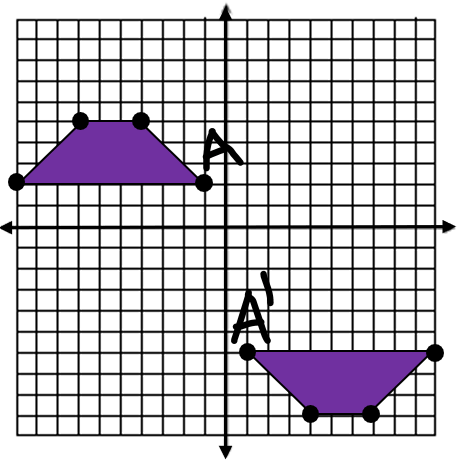Rotating 180 around the point (0, -2)

Click to zoom